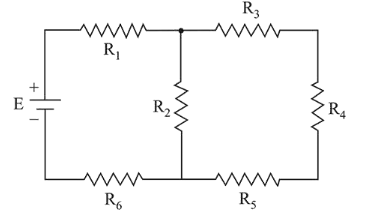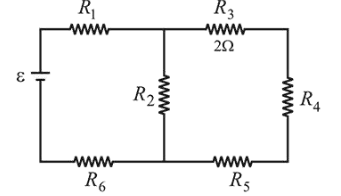# In the figure shown, what is the current (in Ampere) drawn from the battery? You are given :Question:

In the figure shown, what is the current (in Ampere) drawn from the battery? You are given :

$\mathrm{R}_{1}=15 \Omega, \mathrm{R}_{2}=10 \Omega, \mathrm{R}_{3}=20 \Omega, \mathrm{R}_{4}=5 \Omega, \mathrm{R}_{5}=25 \Omega, \mathrm{R}_{6}$

$=30 \Omega, \mathrm{E}=15 \mathrm{~V}$1. (1) $13 / 24$

2. (2) $7 / 18$

3. (3) $9 / 32$

4. (4) $20 / 3$

Correct Option: , 3

Solution:

(3) $R_{3}, R_{4}$ and $R_{5}$ are in series so their equivalent $R=20+5+25=50 \Omega$

This is parallel with $R_{2}$, and so net resistance of the circuit$R_{\mathrm{eq}}=\left(\frac{10 \times 50}{10+50}\right)+15+30=\frac{160}{3} \Omega$

So, $i=\frac{\varepsilon}{R_{\mathrm{eq}}}=\frac{15}{(100 / 3)}=\frac{9}{32} A$